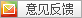PHP 的引用允许用两个变量来指向同一个内容。意思是，当这样做时：

```<?php
\$a =& \$b;
?>
```

Note:

\$a 和 \$b 在这里是完全相同的，这并不是 \$a 指向了 \$b 或者相反，而是 \$a 和 \$b 指向了同一个地方。

Note:

Note:

Example #1 对未定义的变量使用引用

```<?php
function foo(&\$var) { }

foo(\$a); // \$a is "created" and assigned to null

\$b = array();
foo(\$b["b"]);
var_dump(array_key_exists("b", \$b)); // bool(true)

\$c = new StdClass;
foo(\$c->d);
var_dump(property_exists(\$c, "d")); // bool(true)
?>
```

```<?php
\$bar =& new fooclass();
\$foo =& find_var(\$bar);
?>```

Note:

Warning

Example #2 在函数内引用全局变量

```<?php
\$var1 = "Example variable";
\$var2 = "";

function global_references(\$use_globals)
{
global \$var1, \$var2;
if (!\$use_globals) {
\$var2 =& \$var1; // visible only inside the function
} else {
\$GLOBALS["var2"] =& \$var1; // visible also in global context
}
}

global_references(false);
echo "var2 is set to "\$var2"
"; // var2 is set to ""
global_references(true);
echo "var2 is set to "\$var2"
"; // var2 is set to "Example variable"
?>

```

Note:

Example #3 引用与 foreach 语句

```<?php
\$ref = 0;
\$row =& \$ref;
foreach (array(1, 2, 3) as \$row) {
// do something
}
echo \$ref; // 3 - last element of the iterated array
?>

```

```<?php
function foo(&\$var)
{
\$var++;
}

\$a=5;
foo(\$a);
?>

```

```<?php
function foo(&\$var)
{
\$var =& \$GLOBALS["baz"];
}
foo(\$bar);
?>

```

```<?php
function foo(&\$var)
{
\$var++;
}

\$a=5;
foo(\$a);
// \$a is 6 here
?>

```

New 语句，例如 foo(new foobar())

```<?php
function &bar()
{
\$a = 5;
return \$a;
}
foo(bar());
?>

```

```<?php
function bar() // Note the missing &
{
\$a = 5;
return \$a;
}
foo(bar()); // 自 PHP 5.0.5 起导致致命错误
foo(\$a = 5) // 表达式，不是变量
foo(5) // 导致致命错误
?>

```

```<?php
class foo {
public \$value = 42;

public function &getValue() {
return \$this->value;
}
}

\$obj = new foo;
\$myValue = &\$obj->getValue(); // \$myValue is a reference to \$obj->value, which is 42.
\$obj->value = 2;
echo \$myValue;    // prints the new value of \$obj->value, i.e. 2.
?>

```

Note: 和参数传递不同，这里必须在两个地方都用 & 符号——指出返回的是一个引用，而不是通常的一个拷贝，同样也指出 \$myValue 是作为引用的绑定，而不是通常的赋值。

Note: 如果试图这样从函数返回引用：return (\$this->value);，这将不会起作用，因为在试图返回一个表达式的结果而不是一个引用的变量。只能从函数返回引用变量——没别的方法。如果代码试图返回一个动态表达式或 new 运算符的结果，自 PHP 4.4.0 和 PHP 5.1.0 起会发出一条 E_NOTICE 错误。

```<?php
function &test(){
static \$b=0;//申明一个静态变量
\$b=\$b+1;
echo \$b;
return \$b;
}
\$a=test();//这条语句会输出\$b的值为１
\$a=5; \$a=test();//这条语句会输出\$b的值为2
\$a=&test();//这条语句会输出\$b的值为3
\$a=5; \$a=test();//这条语句会输出\$b的值为6
?>

```

\$a=test()方式调用函数，只是将函数的值赋给\$a而已，而\$a做任何改变化，都不会影响到函数中的\$b，而通过\$a=&test()方式调用函数呢, 他的作用是将return \$b中的\$b变量的内存地址与\$a变量的内存地址指向了同一个地方，即产生了相当于这样的效果(\$a=&b;) 所以改变\$a的值，也同时改变了\$b的值，所以在执行了 \$a=&test(); \$a=5; 以后，\$b的值变为了5。

```<?php
\$a = 1;
\$b =& \$a;
unset(\$a);
?>
```

global 引用

```<?php
\$var =& \$GLOBALS["var"];
?>
```

\$this

1:如果有下面的代码

```<?ph
\$a="ABC";
\$b=\$a;
?>

```

其实此时，\$a与\$b都是指向同一内存地址，而并不是\$a与\$b占用不同的内存。

２:如果在上面的代码基础上再加上如下代码

\$a="EFG";
由于\$a与\$b所指向的内存的数据要重新写一次了，此时Zend核心会自动判断　自动为\$b生产一个\$a的数据拷贝，重新申请一块内存进行存储。

相关新闻more

15
04月

21
04月

14
04月

08
04月
设计生涯的转折点在哪里？看看这10位创意人的.7x24小时服务电话:18581389571 传真:023-85725751 免费建站交流群：236412099 139947842(自助建站交流) E-Mail:post@yinxi.net 网站投诉: